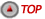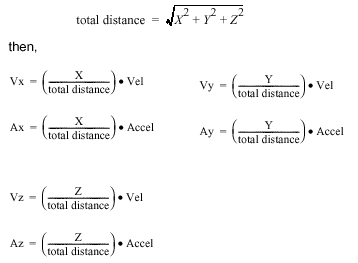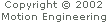### Perform a Point-to-Point Coordinated MoveTo generate a point-to-point coordinated move, you can use mpiMotionStart(...) with either a "trapezoidal" or "S-Curve" type motion. By scaling the Velocities, Accels, and Decels by the ratio of the distances, you can get a point-to-point coordinated move. The coordinated moves will all start and end at the same time. It doesn't matter what the Jerk Percent parameter value is, because the calculated move time is the same for both trapezoidal and S-Curve moves. For example, specifying a vector Vel, Accel, Decel, and a distance X, Y, Z: